This book is archived and will be removed July 6, 2022. Please use the updated version.

Standard Costs and Variances

# 46 Compute and Evaluate Materials Variances

As you’ve learned, direct materials are those materials used in the production of goods that are easily traceable and are a major component of the product. The amount of materials used and the price paid for those materials may differ from the standard costs determined at the beginning of a period. A company can compute these materials variances and, from these calculations, can interpret the results and decide how to address these differences.

Buttering Popcorn

In a movie theater, management uses standards to determine if the proper amount of butter is being used on the popcorn. They train the employees to put two tablespoons of butter on each bag of popcorn, so total butter usage is based on the number of bags of popcorn sold. Therefore, if the theater sells 300 bags of popcorn with two tablespoons of butter on each, the total amount of butter that should be used is 600 tablespoons. Management can then compare the predicted use of 600 tablespoons of butter to the actual amount used. If the actual usage of butter was less than 600, customers may not be happy, because they may feel that they did not get enough butter. If more than 600 tablespoons of butter were used, management would investigate to determine why. Some reasons why more butter was used than expected (unfavorable outcome) would be because of inexperienced workers pouring too much, or the standard was set too low, producing unrealistic expectations that do not satisfy customers.

### Fundamentals of Direct Materials Variances

The direct materials variances measure how efficient the company is at using materials as well as how effective it is at using materials. There are two components to a direct materials variance, the direct materials price variance and the direct materials quantity variance, which both compare the actual price or amount used to the standard amount.

#### Direct Materials Price Variance

The direct materials price variance compares the actual price per unit (pound or yard, for example) of the direct materials to the standard price per unit of direct materials. The formula for direct materials price variance is calculated as: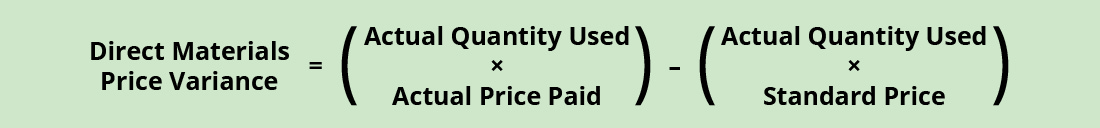Factoring out actual quantity used from both components of the formula, it can be rewritten as:With either of these formulas, the actual quantity used refers to the actual amount of materials used to create one unit of product. The standard price is the expected price paid for materials per unit. The actual price paid is the actual amount paid for materials per unit. If there is no difference between the standard price and the actual price paid, the outcome will be zero, and no price variance exists.

If the actual price paid per unit of material is lower than the standard price per unit, the variance will be a favorable variance. A favorable outcome means you spent less on the purchase of materials than you anticipated. If, however, the actual price paid per unit of material is greater than the standard price per unit, the variance will be unfavorable. An unfavorable outcome means you spent more on the purchase of materials than you anticipated.

The actual price can differ from the standard or expected price because of such factors as supply and demand of the material, increased labor costs to the supplier that are passed along to the customer, or improvements in technology that make the material cheaper. The producer must be aware that the difference between what it expects to happen and what actually happens will affect all of the goods produced using these particular materials. Therefore, the sooner management is aware of a problem, the sooner they can fix it. For that reason, the material price variance is computed at the time of purchase and not when the material is used in production.

Let us consider an example. Connie’s Candy Company produces various types of candies that they sell to retailers. Connie’s Candy establishes a standard price for candy-making materials of ?7.00 per pound. Each box of candy is expected to use 0.25 pounds of candy-making materials. Connie’s Candy found that the actual price of materials was ?6.00 per pound. They still actually use 0.25 pounds of materials to make each box. The direct materials price variance computes as:

$$\text{Direct Materials Price Variance}=\left(?6.00–?7.00\right)\phantom{\rule{0.2em}{0ex}}×\phantom{\rule{0.2em}{0ex}}0.25\phantom{\rule{0.2em}{0ex}}\text{lb.}=?0.25\phantom{\rule{0.2em}{0ex}}\text{or}\phantom{\rule{0.2em}{0ex}}?0.25\phantom{\rule{0.2em}{0ex}}\text{(Favorable)}$$

In this case, the actual price per unit of materials is ?6.00, the standard price per unit of materials is ?7.00, and the actual quantity used is 0.25 pounds. This computes as a favorable outcome. This is a favorable outcome because the actual price for materials was less than the standard price. As a result of this favorable outcome information, the company may consider continuing operations as they exist, or could change future budget projections to reflect higher profit margins, among other things.

Let us take the same example except now the actual price for candy-making materials is ?9.00 per pound. The direct materials price variance computes as:

$$\text{Direct Materials Price Variance}=\left(?9.00–?7.00\right)\phantom{\rule{0.2em}{0ex}}×\phantom{\rule{0.2em}{0ex}}0.25\phantom{\rule{0.2em}{0ex}}\text{lbs.}=?0.50\phantom{\rule{0.2em}{0ex}}\text{or}\phantom{\rule{0.2em}{0ex}}?0.50\phantom{\rule{0.2em}{0ex}}\text{(Unfavorable)}$$

In this case, the actual price per unit of materials is ?9.00, the standard price per unit of materials is ?7.00, and the actual quantity used is 0.25 pounds. This computes as an unfavorable outcome. This is an unfavorable outcome because the actual price for materials was more than the standard price. As a result of this unfavorable outcome information, the company may consider using cheaper materials, changing suppliers, or increasing prices to cover costs.

Another element this company and others must consider is a direct materials quantity variance.

Don’t “Skirt” the Issue

You run a fabric store and order materials through a supplier. At the end of the month, you review your materials cost and discover that your direct materials price and quantity variances produced unfavorable results. What could be attributed to these unfavorable outcomes? How would these unfavorable outcomes impact the total direct materials variance?

#### Direct Materials Quantity Variance

The direct materials quantity variance compares the actual quantity of materials used to the standard materials that were expected to be used to make the actual units produced. The variance is calculated using this formula: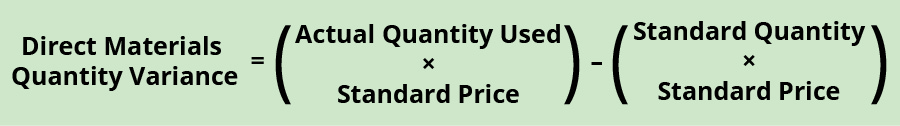Factoring out standard price from both components of the formula, it can be rewritten as:With either of these formulas, the actual quantity used refers to the actual amount of materials used at the actual production output. The standard price is the expected price paid for materials per unit. The standard quantity is the expected amount of materials used at the actual production output. If there is no difference between the actual quantity used and the standard quantity, the outcome will be zero, and no variance exists.

If the actual quantity of materials used is less than the standard quantity used at the actual production output level, the variance will be a favorable variance. A favorable outcome means you used fewer materials than anticipated, to make the actual number of production units. If, however, the actual quantity of materials used is greater than the standard quantity used at the actual production output level, the variance will be unfavorable. An unfavorable outcome means you used more materials than anticipated to make the actual number of production units.

The actual quantity used can differ from the standard quantity because of improved efficiencies in production, carelessness or inefficiencies in production, or poor estimation when creating the standard usage.

Consider the previous example with Connie’s Candy Company. Connie’s Candy established a standard price for candy-making materials of ?7.00 per pound. Each box of candy is expected to use 0.25 pounds of candy-making materials. Connie’s Candy found that the actual quantity of candy-making materials used to produce one box of candy was 0.20 per pound. The direct materials quantity variance computes as:

$$\text{Direct Materials Quantity Variance}=\left(0.20\phantom{\rule{0.2em}{0ex}}\text{lb.}–0.25\phantom{\rule{0.2em}{0ex}}\text{lb.}\right)\phantom{\rule{0.2em}{0ex}}×\phantom{\rule{0.2em}{0ex}}?7.00=–?0.35\phantom{\rule{0.2em}{0ex}}\text{or}\phantom{\rule{0.2em}{0ex}}?0.35\phantom{\rule{0.2em}{0ex}}\text{(Favorable)}$$

In this case, the actual quantity of materials used is 0.20 pounds, the standard price per unit of materials is ?7.00, and the standard quantity used is 0.25 pounds. This computes as a favorable outcome. This is a favorable outcome because the actual quantity of materials used was less than the standard quantity expected at the actual production output level. As a result of this favorable outcome information, the company may consider continuing operations as they exist, or could change future budget projections to reflect higher profit margins, among other things.

Let us take the same example except now the actual quantity of candy-making materials used to produce one box of candy was 0.50 per pound. The direct materials quantity variance computes as:

$$\text{Direct Materials Quantity Variance}=\left(0.50\phantom{\rule{0.2em}{0ex}}\text{lb.}–0.25\phantom{\rule{0.2em}{0ex}}\text{lb.}\right)\phantom{\rule{0.2em}{0ex}}×\phantom{\rule{0.2em}{0ex}}?7.00=?1.75\phantom{\rule{0.2em}{0ex}}\text{or}\phantom{\rule{0.2em}{0ex}}?1.75\phantom{\rule{0.2em}{0ex}}\text{(Unfavorable)}$$

In this case, the actual quantity of materials used is 0.50 pounds, the standard price per unit of materials is ?7.00, and the standard quantity used is 0.25 pounds. This computes as an unfavorable outcome. This is an unfavorable outcome because the actual quantity of materials used was more than the standard quantity expected at the actual production output level. As a result of this unfavorable outcome information, the company may consider retraining workers to reduce waste or change their production process to decrease materials needs per box.

The combination of the two variances can produce one overall total direct materials cost variance.

### Total Direct Materials Cost Variance

When a company makes a product and compares the actual materials cost to the standard materials cost, the result is the total direct materials cost variance.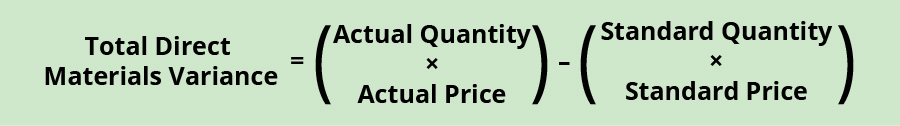An unfavorable outcome means the actual costs related to materials were more than the expected (standard) costs. If the outcome is a favorable outcome, this means the actual costs related to materials are less than the expected (standard) costs.

The total direct materials cost variance is also found by combining the direct materials price variance and the direct materials quantity variance. By showing the total materials variance as the sum of the two components, management can better analyze the two variances and enhance decision-making.

(Figure) shows the connection between the direct materials price variance and direct materials quantity variance to total direct materials cost variance.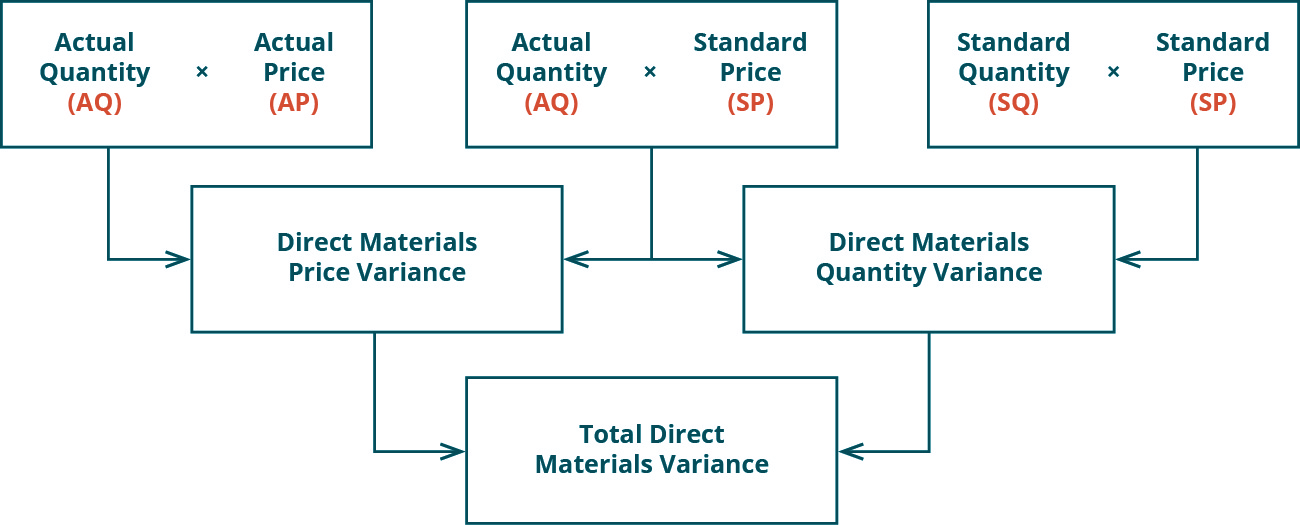For example, Connie’s Candy Company expects to pay ?7.00 per pound for candy-making materials but actually pays ?9.00 per pound. The company expected to use 0.25 pounds of materials per box but actually used 0.50 per box. The total direct materials variance is computed as:

$$\text{Total Direct Materials Variance}=\left(0.50\phantom{\rule{0.2em}{0ex}}\text{lbs.}\phantom{\rule{0.2em}{0ex}}×\phantom{\rule{0.2em}{0ex}}?9.00\right)–\left(0.25\phantom{\rule{0.2em}{0ex}}\text{lbs.}\phantom{\rule{0.2em}{0ex}}×\phantom{\rule{0.2em}{0ex}}?7.00\right)=?4.50–?1.75=?2.75\phantom{\rule{0.2em}{0ex}}\text{(Unfavorable)}$$

In this case, two elements contribute to the unfavorable outcome. Connie’s Candy paid ?2.00 per pound more for materials than expected and used 0.25 pounds more of materials than expected to make one box of candy.

The same calculation is shown using the outcomes of the direct materials price and quantity variances.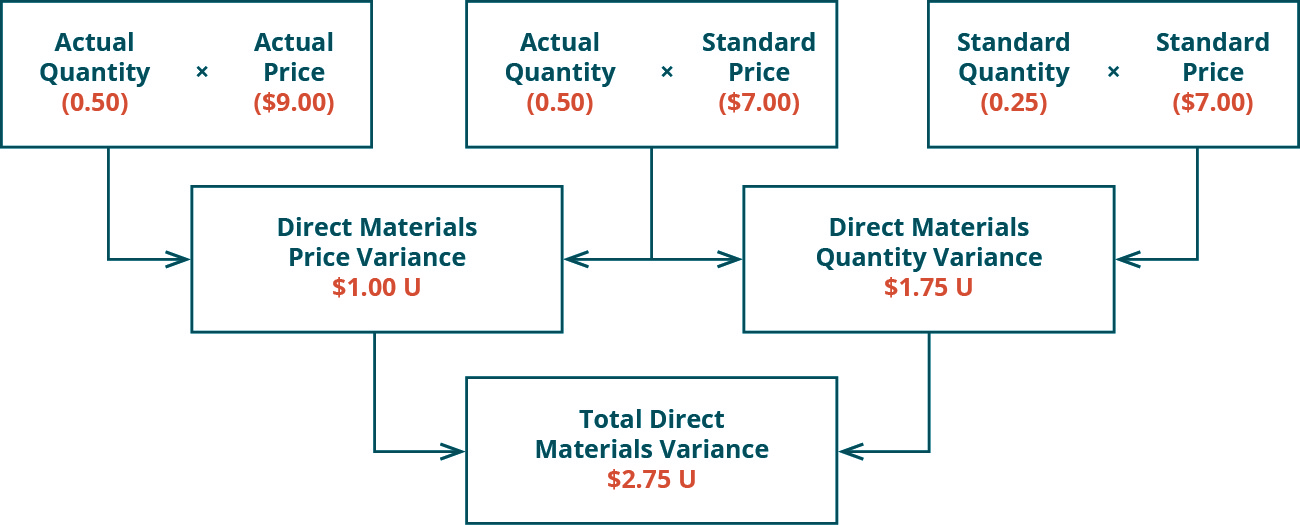As with the interpretations for the materials price and quantity variances, the company would review the individual components contributing to the overall unfavorable outcome for the total direct materials variance, and possibly make changes to production elements as a result.

Sweet and Fresh Shampoo Materials

Biglow Company makes a hair shampoo called Sweet and Fresh. Each bottle has a standard material cost of 8 ounces at ?0.85 per ounce. During May, Biglow manufactured 11,000 bottles. They bought 89,000 ounces of material at a cost of ?74,760. All 89,000 ounces were used to make the 11,000 bottles. Calculate the material price variance and the material quantity variance.

Solution

Actual price per pound: 74,760/89,000 = ?0.84

Material price variance: 89,000 × (0.84 − 0.85) = ?890 favorable

Material quantity variance: 0.85 × (89,000 – 88,000) = ?850 unfavorable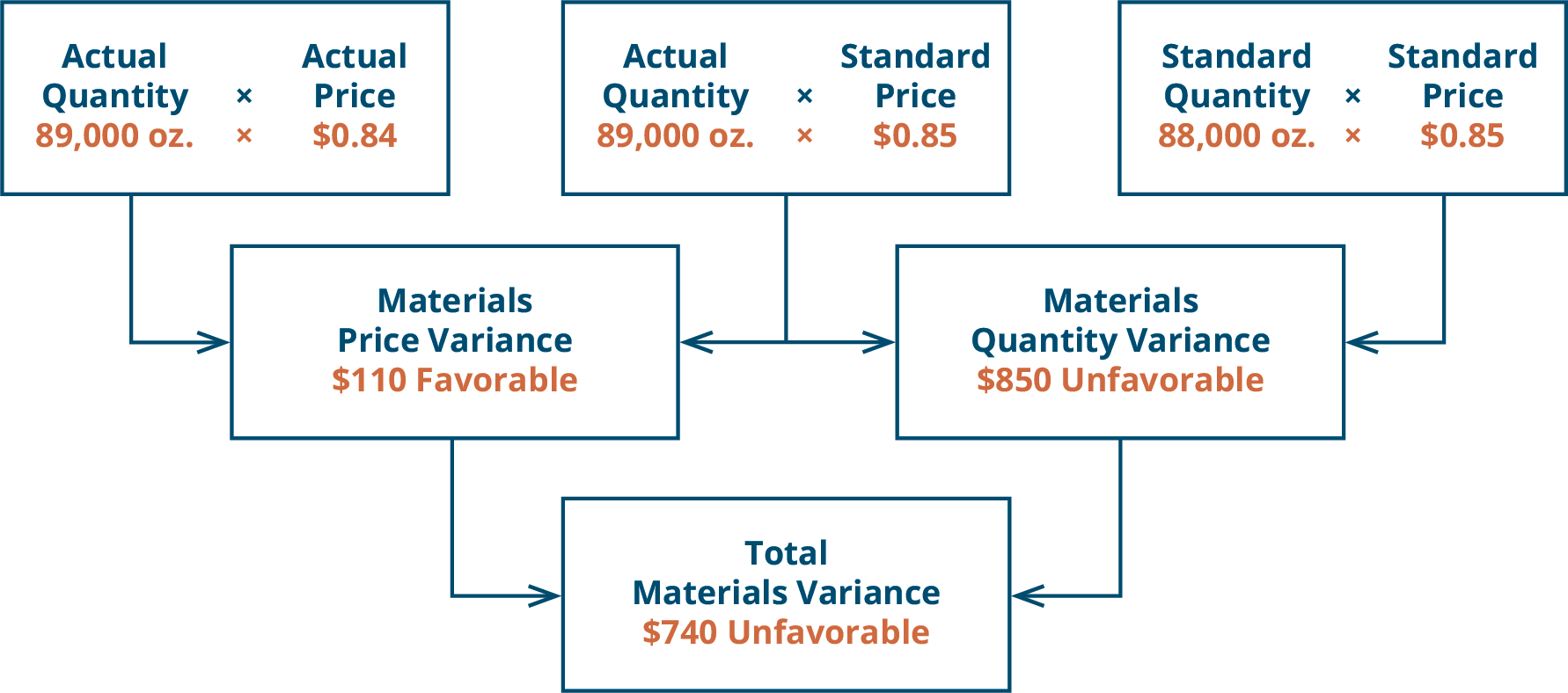### Key Concepts and Summary

• There are two components to material variances: the direct materials price variance and the direct materials quantity variance.
• The direct materials price variance is caused by paying too much or too little for material.
• The direct materials quantity variance is caused by using too much or too little material.

(Figure)What are some possible reasons for a material price variance?

1. substandard material
2. labor rate increases
3. labor rate decreases
4. labor efficiency

(Figure)When is the material price variance unfavorable?

1. when the actual quantity used is greater than the standard quantity
2. when the actual quantity used is less than the standard quantity
3. when the actual price paid is greater than the standard price
4. when the actual price is less than the standard price

C

(Figure)When is the material price variance favorable?

1. when the actual quantity used is greater than the standard quantity
2. when the actual quantity used is less than the standard quantity
3. when the actual price paid is greater than the standard price
4. when the actual price is less than the standard price

(Figure)What are some reasons for a material quantity variance?

1. building rental charges increase
2. labor rate decreases
3. more qualified workers
4. labor efficiency increases

C

(Figure)When is the material quantity variance favorable?

1. when the actual quantity used is greater than the standard quantity
2. when the actual quantity used is less than the standard quantity
3. when the actual price paid is greater than the standard price
4. when the actual price is less than the standard price

(Figure)When is the material quantity unfavorable?

1. when the actual quantity used is greater than the standard quantity
2. when the actual quantity used is less than the standard quantity
3. when the actual price paid is greater than the standard price
4. when the actual price is less than the standard price

A

(Figure)What causes the material price variance?

Paying more or less than the standard price

(Figure)What causes the material quantity variance?

(Figure)What are some possible causes of a material price variance?

(Figure)What are some possible causes of a material quantity variance?

(Figure)Sitka Industries uses a cost system that carries direct materials inventory at a standard cost. The controller has established these standards for one ladder (unit):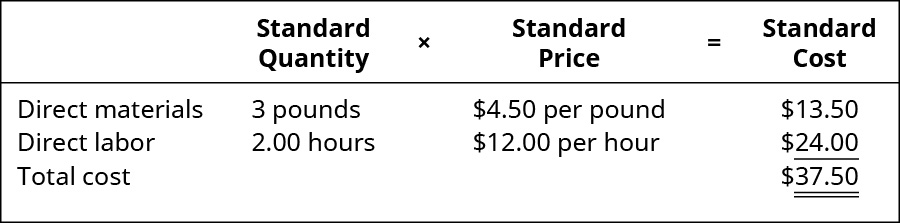Sitka Industries made 3,000 ladders in July and used 8,800 pounds of material to make these units. Smith Industries bought 15,500 pounds of material in the current period. There was a ?250 unfavorable direct materials price variance.

1. How much in total did Sitka pay for the 15,500 pounds?
2. What is the direct materials quantity variance?
3. What is the total direct material cost variance?
4. What if 9,500 pounds were used to make these ladders, what would be the direct materials quantity variance?
5. If there was a ?340 favorable direct materials price variance, how much did Sitka pay for the 15,500 pounds of material?

(Figure)Use the information provided to answer the questions.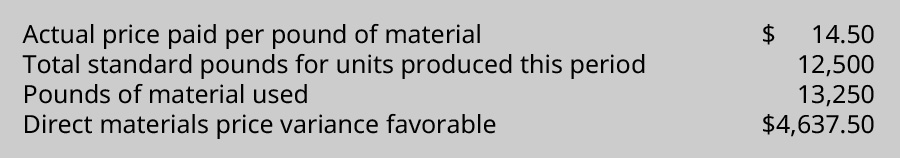All material purchased was used in production.

1. What is the standard price paid for materials?
2. What is the direct materials quantity variance?
3. What is the total direct materials cost variance?
4. If the direct materials price variance was unfavorable, what would be the standard price?

(Figure)Dog Bone Bakery, which bakes dog treats, makes a special biscuit for dogs. Each biscuit uses 0.75 cup of pure semolina flour. They buy 4,000 cups of flour at ?0.55 per cup. They use 3,550 cups of flour to make 4,750 biscuits. The standard cost per cup of flour is ?0.53.

1. What are the direct materials price variance, the direct materials quantity variances, and the total direct materials cost variance?
2. What is the standard cost per biscuit for the semolina flour?

(Figure)Smith Industries uses a cost system that carries direct materials inventory at a standard cost. The controller has established these standards for the cost of one basket (unit):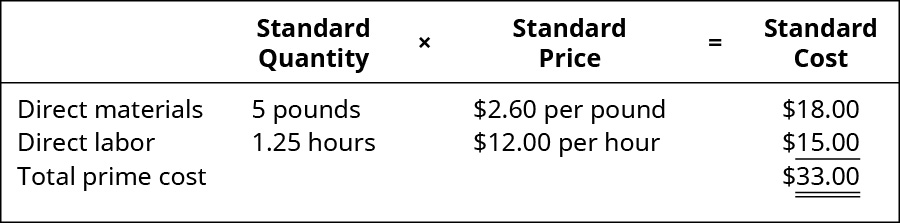Smith Industries made 3,000 baskets in July and used 15,500 pounds of material to make these units. Smith Industries paid ?39,370 for the 15,500 pounds of material.

1. What was the direct materials price variance for July?
2. What was the direct materials quantity variance for July?
3. What is the total direct materials cost variance?
4. If Smith Industries used 15,750 pounds to make the baskets, what would be the direct materials quantity variance?

(Figure)Lizbeth, Inc., makes ice cream. The toffee coffee ice cream takes 4 quarts of cream, 3 cups of sugar, 2 tablespoons of toffee flavoring, and 1.5 tablespoons of coffee flavoring per gallon. The standard prices are ?2.00 per quart of cream, ?0.40 per cup of sugar, ?0.50 per tablespoon of toffee flavoring, and ?0.75 per tablespoon of coffee flavoring.

1. What is the standard material cost for a gallon of toffee coffee ice cream?
2. If Lizbeth makes 35 gallons of toffee coffee ice cream, how much of each of the ingredients should she use?
3. If Lizbeth uses 105 quarts of cream to make 25 gallons of ice cream, what would be the cream (direct materials) quantity variance?
4. If Lizbeth uses 45 tablespoons of toffee flavoring to make 25 gallons of ice cream, what would be the toffee flavoring (direct materials) quantity variance?

(Figure)Woodpecker manufactures sawmill equipment. They use a standard costing system and recognize material price variance at the time of material purchases. They use carbide to make the teeth on their band-saw blades. They received an order for 250 band-saw blades, but they did not have any carbide in stock. They purchased 3,500 pounds of carbide for ?14,875 but should have spent ?16,275. Each saw blade has a standard carbide direct materials quantity of 7.8 pounds.

1. If they used 8 pounds per blade, what would be the direct materials quantity variance?
2. If they used 7.5 pounds per blade, what would be the direct materials quantity variance?
3. Compute the direct materials price variance based on 7.5 pounds of carbide per blade actually used.

(Figure)April Industries employs a standard costing system in the manufacturing of its sole product, a park bench. They purchased 60,000 feet of raw material for ?300,000, and it takes 5 feet of raw materials to produce one park bench. In August, the company produced 10,000 park benches. The standard cost for material output was ?100,000, and there was an unfavorable direct materials quantity variance of ?6,000.

1. What is April Industries’ standard price for one unit of material?
2. What was the total number of units of material used to produce the August output?
3. What was the direct materials price variance for August?

(Figure)Ed Co. manufactures two types of O rings, large and small. Both rings use the same material but require different amounts. Standard materials for both are shown.

 Large Small Rubber 3 feet at ?0.25 per foot 1.25 feet at ?0.25 per foot Connector 1 at ?0.03 1 at ?0.03

At the beginning of the month, Ed Co. bought 25,000 feet of rubber for ?6,875. The company made 3,000 large O rings and 4,000 small O rings. The company used 14,500 feet of rubber.

1. What are the direct materials price variance, the direct materials quantity variance, and the total direct materials cost variance?
2. If they bought 10,000 connectors costing ?310, what would the direct materials price variance be for the connectors?
3. If there was an unfavorable direct materials price variance of ?125, how much did they pay per foot for the rubber?

(Figure)The Whizbang Company makes a special type of toy. Each top takes 6 ounces of a special material that costs ?3 per ounce. Whizbang bought 4,000 ounces of the material at a cost of ?11,300. They used 3,400 ounces to make 534 toys. Compute the direct materials price variance, the direct materials quantity variance, and the total direct materials cost variance.

(Figure)A company bought 45,000 pounds of plastic pellets to make DVDs at a cost of ?9,900. The standard cost per pound for the pellets is 20.5 cents. Some of these pellets were used in three jobs. The first job called for 7,500 pounds but used 7,250 pounds. The second job called for 8,800 pounds but used 9,000 pounds. The third job called for 2,300 pounds but used 2,250 pounds. Compute the direct materials price variance and the direct materials quantity variance for each job and in total. Why would you want to calculate the direct materials quantity variance for each job?

(Figure)Illinois Company is a medium-sized company that makes dresses. During the month of June, 8,575 dresses were made. All material purchases were used to make the dresses. The company had this information: standard per dress of 6 yards of material at ?6.20 per yard. The actual quantity was 52,000 yards at a cost of ?325,520. Compute the direct materials price variance, the direct materials quantity variance, and the total direct materials cost variance.

(Figure)Corolla Manufacturing has a standard cost for steel of ?20 per pound for a product that uses 4 pounds of steel. During September, Corolla purchased and used 4,200 pounds of steel to make 1,040 units. They paid ?20.75 per pound for the steel. Compute the direct materials price variance, the direct materials quantity variance, and the total direct materials cost variance for the month of September. What would change if Corolla had made 2,200 units?

(Figure)You started your own construction business and need to determine the cost of materials used to build one house, and how many materials you will need to do so.

• Where would you begin to determine the standard price and quantity needs to build one house?
• What would produce a difference between the standard cost to build a house and the actual cost? What would cause a favorable outcome? What would cause an unfavorable outcome?
• What action might your company take if you had an unfavorable total direct materials cost variance?

### Glossary

direct materials price variance
difference between the actual price paid per unit for materials and what should have been paid per the standards
direct materials quantity variance
difference between the actual quantity of materials used and the standard materials that were expected to be used to make the actual units produced
direct materials variance
difference between the actual price or amount used and the standard amount
total direct materials cost variance
difference between actual materials cost and standard materials cost